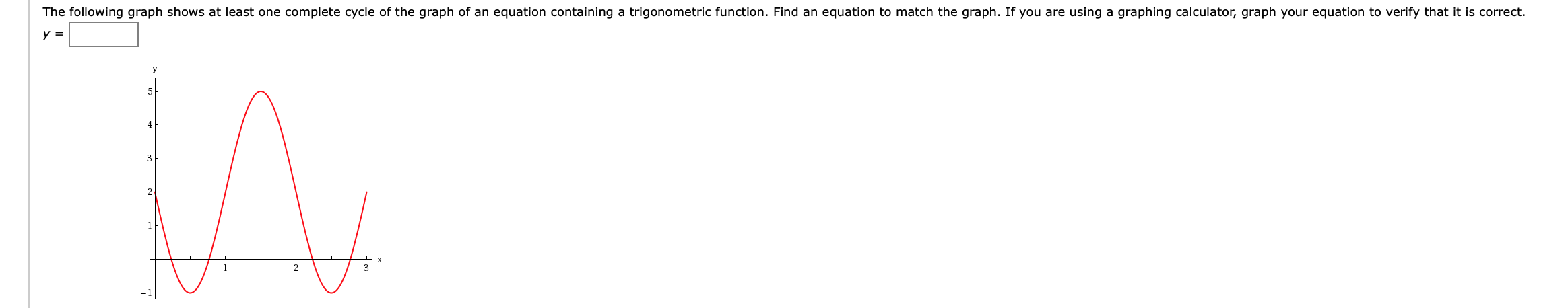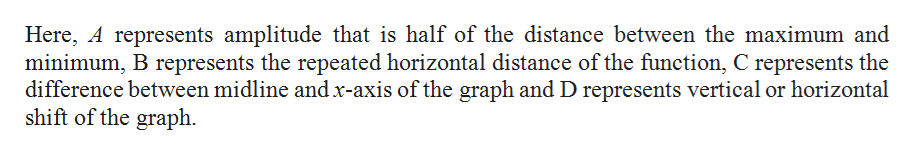# The following graph shows at least one complete cycle of the graph of an equation containing a trigonometric function. Find an equation to match the graph. If you are using a graphing calculator, graph your equation to verify that it is correcty =y4223

Question
3 viewshelp_outlineImage TranscriptioncloseThe following graph shows at least one complete cycle of the graph of an equation containing a trigonometric function. Find an equation to match the graph. If you are using a graphing calculator, graph your equation to verify that it is correct y = y 4 2 2 3 fullscreen
check_circle

Step 1

The equation Y=Asin(Bx+D)...help_outlineImage TranscriptioncloseHere, A represents amplitude that is half of the distance between the maximum and minimum, B represents the repeated horizontal distance of the function, C represents the difference between midline andx-axis of the graph and D represents vertical or horizontal shift of the graph fullscreen

### Want to see the full answer?

See Solution

#### Want to see this answer and more?

Solutions are written by subject experts who are available 24/7. Questions are typically answered within 1 hour.*

See Solution
*Response times may vary by subject and question.
Tagged in

### Other